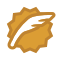# 程序员的数学--用贝叶斯定理来推断一个案子

### 什么是贝叶斯定理？

$P\left(H\mathrm{\mid }E\right)=P\left(H\right)\ast P\left(E\mathrm{\mid }H\right)\mathrm{/}P\left(E\right)$P(H∣E)=P(H)∗P(E∣H)/P(E)

### 条件概率

P(E|H) 是条件概率，当事件 H 发生时，事件 E 也发生的概率是多少

#### 标准似然度 1

$P\left(E\mathrm{\mid }H\right)\mathrm{/}P\left(E\right)$P(E∣H)/P(E)

### 后验概率

P(H|E) 是条件概率，但因为标准似然度的原因，它被称为后验概率。
$P\left(\mathrm{后}\mathrm{验}\right)=\mathrm{标}\mathrm{准}\mathrm{似}\mathrm{然}\mathrm{度}\ast P\mathrm{（}\mathrm{先}\mathrm{验}\right)$P(后验)=标准似然度∗P（先验)

### 贝叶斯推理尝试

#### 1. 给假设概率

$P\left(H\right)=0.6$P(H)=0.6

#### 2. 观察论证

$P\left(E\right)=P\left(E\mathrm{\mid }H\right)\ast P\left(H\right)+P\left(E\mathrm{\mid }A\right)\ast P\mathrm{（}A\mathrm{）}$P(E)=P(E∣H)∗P(H)+P(E∣A)∗P（A）

P(E) 的意义是一个人做猥琐动作的概率，包括了正人君子做猥琐动作的概率和猥琐男做猥琐动作的概率。

$P\left(E\mathrm{\mid }H\right)=5\mathrm{/}60=0.083$P(E∣H)=5/60=0.083

$P\left(E\mathrm{\mid }A\right)=36\mathrm{/}40=0.9$P(E∣A)=36/40=0.9

$P\left(E\right)=P\left(E\mathrm{\mid }H\right)\ast P\left(H\right)+P\left(E\mathrm{\mid }A\right)\ast P\mathrm{（}A\mathrm{）}=0.083\ast 0.6+0.9\ast 0.4=0.05+0.36=0.41$P(E)=P(E∣H)∗P(H)+P(E∣A)∗P（A）=0.083∗0.6+0.9∗0.4=0.05+0.36=0.41

$P\left(H\mathrm{\mid }E\right)=P\left(H\right)\ast P\left(E\mathrm{\mid }H\right)\mathrm{/}P\left(E\right)=0.6\ast 0.083\mathrm{/}0.41=0.12$P(H∣E)=P(H)∗P(E∣H)/P(E)=0.6∗0.083/0.41=0.12

### 数学题目练习

A 代表男孩子，所以 P(A) 为 0.45

B 代表女孩子，所以P(B) 为 0.55

E 代表扔东西，P(E|A) = 0.6,P(E|B)=0.4，所以：
$P\left(E\right)=P\left(E\mathrm{\mid }A\right)\ast P\left(A\right)+P\left(E\mathrm{\mid }B\right)\ast P\left(B\right)=0.6\ast 0.45+0.4\ast 0.55=0.49$P(E)=P(E∣A)∗P(A)+P(E∣B)∗P(B)=0.6∗0.45+0.4∗0.55=0.49

$P\left(A\mathrm{\mid }E\right)=P\left(A\right)\ast P\left(E\mathrm{\mid }A\right)\mathrm{/}P\left(E\right)=0.45\ast 0.6\mathrm{/}0.49=0.55$P(A∣E)=P(A)∗P(E∣A)/P(E)=0.45∗0.6/0.49=0.55

### 贝叶斯定理的影响因子

$P\left(H\mathrm{\mid }E\right)=P\left(H\right)\ast P\left(E\mathrm{\mid }H\right)\mathrm{/}P\left(E\right)$P(H∣E)=P(H)∗P(E∣H)/P(E)

$P\left(E\right)=P\left(E\mathrm{\mid }H\right)\ast P\left(H\right)+P\left(E\mathrm{\mid }A\right)\ast P\mathrm{（}A\mathrm{）}$P(E)=P(E∣H)∗P(H)+P(E∣A)∗P（A）

P(H)P(A)P(E|H)P(E|A)

### 参考frank909CSDN认证博客专家CV(computer vision)爱阅读的程序员，专注于技术思考和分享。关注架构设计、Android 开发、AI、数学、自动驾驶领域，个人公号:Frankcall